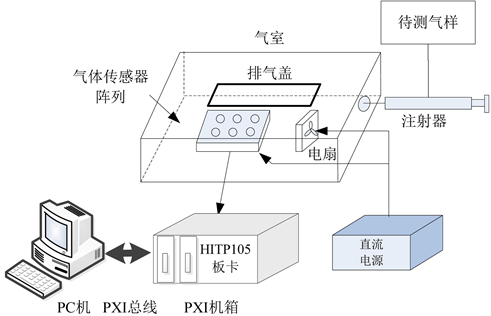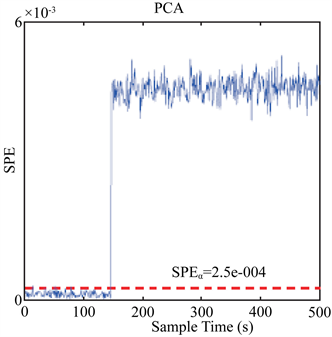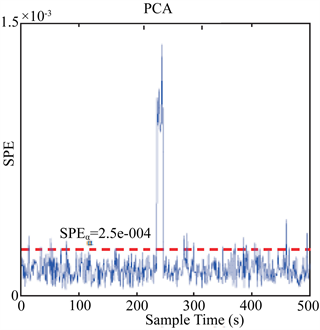﻿ 一种面向多传感器系统的故障检测与隔离算法

# 一种面向多传感器系统的故障检测与隔离算法A Fault Detection and Isolation Algorithm for Multi-Sensor System

Abstract: Fault detection and isolation technology is one of the core research contents of self-identified sensors. Aiming at the problem of low fault detection and isolation accuracy in the abnormal state monitoring process of multi-sensor system, this paper proposes a fault detection and separation algorithm based on principal component analysis (PCA) adaptive reconstruction technology. The method uses the normal data of the multi-sensor system to establish the PCA model, and monitors the multi-channel signals of the sensor system in real time through the SPE statistics. Once the fault occurs, the value of the SPE statistic will be significantly improved to achieve the detection of the sensor fault. In order to further isolate the fault sensor, the SPE statistic adaptive reconstruction technology is used to locate the fault sensor. Simulation experiments show that the fault and isolation algorithm of multi-sensor system proposed in this paper can effectively realize fault detection and isolation of multi-sensor system, and has high accuracy.

1. 引言

2. 基于主成分分析的故障检测

$X=\stackrel{^}{X}+\stackrel{˜}{X}=\stackrel{^}{T}{\stackrel{^}{P}}^{\text{T}}+E={\stackrel{^}{t}}_{1}{\stackrel{^}{p}}_{1}^{\text{T}}+{\stackrel{^}{t}}_{2}{\stackrel{^}{p}}_{2}^{\text{T}}+\cdots +{\stackrel{^}{t}}_{k}{\stackrel{^}{p}}_{k}^{\text{T}}+E$ (1)

$\Sigma =\frac{1}{n-1}{X}^{\text{T}}X$ (2)

$\Sigma =U\Lambda {U}^{\text{T}}$ (3)

$CPV\left(k\right)=\frac{\underset{i=1}{\overset{k}{\sum }}{\lambda }_{i}}{trace\left(\Sigma \right)}×100%$ (4)

$C=\stackrel{^}{P}{\stackrel{^}{P}}^{\text{T}}，\stackrel{˜}{C}=\stackrel{˜}{P}{\stackrel{˜}{P}}^{\text{T}}=\left(I-C\right)$ (5)

PCA是一种有效的系统故障检测工具，主要通过平方预测误差SPE和Hotelling’s T2统计指标实现，定义如下：

$\text{SPE}={‖\stackrel{˜}{x}‖}^{2}=x\stackrel{˜}{C}{\stackrel{˜}{C}}^{\text{T}}{x}^{\text{T}}=x\stackrel{˜}{C}{x}^{\text{T}}$ (6)

${\text{SPE}}_{\alpha }={\theta }_{1}{\left[1+\frac{{h}_{0}{C}_{\alpha }\sqrt{2{\theta }_{2}}}{{\theta }_{1}}+\frac{{\theta }_{2}{h}_{0}\left({h}_{0}-1\right)}{{\theta }_{1}^{2}}\right]}^{\frac{1}{{h}_{0}}}$ (7)

${\text{T}}^{2}={x}^{\text{T}}\stackrel{^}{P}{\Lambda }_{k}^{-1}{\stackrel{^}{P}}^{\text{T}}x={x}^{\text{T}}Dx$ (8)

${\text{T}}_{\alpha }=\frac{\left({n}^{2}-1\right)k}{n\left(n-k\right)}{F}_{\alpha }\left(k,n-k\right)$ (9)

PCA故障检测模型是通过正常运行状态下的数据建立的，在系统正常情况下，SPE和T2统计量都小于各自的控制限。一旦系统发生故障，将导致SPE和T2统计量发生突变，超过控制限 ${\text{SPE}}_{\alpha }$${\text{T}}_{\alpha }^{2}$ ，从而实现故障检测。

3. 基于自适应重构的故障隔离

$\stackrel{˜}{x}\left(t\right)=x\left(t\right)-\underset{i=1}{\overset{p}{\sum }}{f}_{{i}_{j}}{\xi }_{{i}_{j}}$ (10)

$\mathrm{arg}\underset{p,\Xi ,F}{\mathrm{min}}\text{SPE}\left(\stackrel{˜}{x}\left(t\right)\right)$ (11)

$\text{SPE}\left({x}_{i}\right)={‖{\stackrel{˜}{x}}_{i}‖}^{2}={‖\stackrel{˜}{x}-{\stackrel{˜}{\Xi }}_{i}{f}_{i}‖}^{2}$ (12)

${f}_{i}={\stackrel{˜}{\Xi }}_{i}^{+}\stackrel{˜}{x}={\stackrel{˜}{\Xi }}_{i}^{+}x$ (13)

${x}_{i}=x-{\Xi }_{i}{\stackrel{˜}{\Xi }}_{i}^{+}x=\left(I-{\Xi }_{i}{\stackrel{˜}{\Xi }}_{i}^{+}\right)x$ (14)

${\stackrel{˜}{x}}_{i}=\left(I-{\stackrel{˜}{\Xi }}_{i}{\stackrel{˜}{\Xi }}_{i}^{+}\right)\stackrel{˜}{x}$ (15)

$\text{SPE}\left({x}_{i}\right)={‖{\stackrel{˜}{x}}_{i}‖}^{2}={‖{\stackrel{˜}{x}}^{*}‖}^{2}$ (16)

4. 算法流程Figure 1. Flow chart of fault detection and isolation algorithm for multi-sensor system

5. 实验

5.1. 多传感器实验系统Table 1. Selection of MOS Gas Sensor ArrayFigure 2. Structure diagram of multi-sensor experimental system

5.2. 实验结果Figure 3. The result of failure detection under bias failureFigure 4. The result of failure detection under impacted failureTable 2. Detection rate of PCA failure detection model under different failure typesTable 3. Accuracy of multiple failure isolation with different fault isolation methods

6. 结论

 Henry, M.P. and Clarke, D.W. (1993) The Self-Validating Sensor: Rationale, Definitions and Examples. Control Engineering Practice, 1, 585-610.
https://doi.org/10.1016/0967-0661(93)91382-7

 Shen, Z. and Wang, Q. (2012) Failure Detection, Isolation, and Recovery of Multifunctional Self-Validating Sensor. IEEE Transactions on Instrumentation and Measurement, 61, 3351-3362.
https://doi.org/10.1109/TIM.2012.2205509

 Shen, Z. and Wang, Q. (2013) Data Validation and Validated Uncertainty Esti-mation of Multifunctional Self-Validating Sensors. IEEE Transactions on Instrumentation and Measurement, 62, 2082-2092.
https://doi.org/10.1109/TIM.2013.2253912

 Hwang, I., Kim, S., Kim, Y., et al. (2010) A Survey of Fault Detection, Isolation, and Reconfiguration Methods. IEEE Transactions on Control Systems Technology, 18, 636-653.
https://doi.org/10.1109/TCST.2009.2026285

 Qin, S.J. (2012) Survey on Data-Driven Industrial Process Monitoring and Diagnosis. Annual Reviews in Control, 36, 220-234.
https://doi.org/10.1016/j.arcontrol.2012.09.004

 Weighell, M., Martin, E.B. and Morris, A.J. (2001) The Statistical Monitoring of a Complex Manufacturing Process. Journal of Applied Statistics, 28, 409-425.
https://doi.org/10.1080/02664760120034144

Top